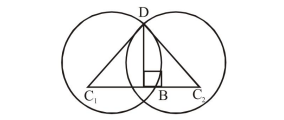# Let the latus ractum of the parabola

Question:

Let the latus ractum of the parabola $y^{2}=4 x$ be the common chord to the circles $C_{1}$ and $C_{2}$ each

of them having radius $2 \sqrt{5}$. Then, the distance

between the centres of the circles $\mathrm{C}_{1}$ and $\mathrm{C}_{2}$ is:

1. 8

2. $4 \sqrt{5}$

3. 12

4. $8 \sqrt{5}$

Correct Option: 1,

Solution:

Length of latus rectum $=4$$\mathrm{DB}=2$

$C_{1} B=\sqrt{\left(C_{1} D\right)^{2}-(D B)^{2}}=4$

$\mathrm{C}_{1} \mathrm{C}_{2}=8$